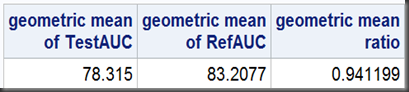# Statistical Notes (1): Geometric Mean and Geometric Mean Ratio

Just read since SAS 9.2, the TTEST procedure also natively supports Equivalence Test by simply adding a TOST option (Two one-sided tests). In a example, TTEST procedure reports a geometric mean as 0.9412, which is the geometric mean of a ratio, TestAUC/RefAUC. It can also be calculated manually by

proc sql;
select exp(mean(log(TestAUC/RefAUC)))
“geometric mean of TestAUC/RefAUC”
from auc;
quit;Actually this kind of geometric mean (of a ratio) is more often called geometric mean ratio(a ratio of two geometric means, in this case, geometric mean of TestAUC and geometric mean of RefAUC). Similarly,

proc sql;
select exp(mean(log(TestAUC))) as gmTest
“geometric mean of TestAUC”,
exp(mean(log(RefAUC)))  as gmRef
“geometric mean of RefAUC” ,
CALCULATED  gmTest / CALCULATED  gmRef as gmr
“geometric mean ratio”
from auc;
quit;78.31583.2077 (the ratio of two geometric means) simply gets 0.941199 (geometric mean ratio), which can be also derived by calculating the geometric mean of a ratio (see above). You can prove it mathematically by playing some log-transformations:

geomean(A) / geomean(B) = geomean(A / B)

In vaccine trials (I worked before) where the interested values are antibody titers,  the geometric mean is also called Geometric Mean Titer (GMT), while geometric mean ratio  referred as Geometric Mean Titer Ratio (GMTR, also named “n-fold rise”). Both GMT and GMTR are wildly presented in statistical analysis reports.

Usually, AUC data mentioned above (Area Under Curve, which is based on blood levels) and antibody titer values are log-normal distributed(source data, noted as X), which means, if you take a log-transformation against X, namely Y=log(X), then Y follows the normal distribution (then Y has good properties to perform further statistical analysis). Suppose two treatment groups available, 1 and 2.

Few extremely important conclusions, just as the codes above suggested, exp[mean(Y)]=exp{mean[log(X)]} is just geometric mean of X, and the geometric mean ratio, geomean (X1) / geomean(X2), simply equals exp[mean(Y1) – mean(Y2)]—This is the mathematical equivalence of ratio and difference.

Back to the AUC example, the FDA endorsed equivalence bounds offered,  0.8 and 1.25, which are both ratio values. So, in the analysis, the original values are transformed to geometric mean ratio (0.9412 with 90% limit of [0.8634 , 1.0260]) to compare with the predefined bounds. For details of equivalence testing, I may extend it into a separated note.

# SAS Notes

In SAS/Base, GEOMEAN function is used to calculate the geometric mean. It takes a list of values as argument, which means, it can only perform on rows, but not on columns. So, if need the geometric mean of a variable, TestAUC for example, you should transpose the rows to columns or similarly, use a array:

/1. transpose/
proc transpose data=auc (keep=TestAUC) out=test prefix=t;
var TestAUC;
run;

data null;
set test;
gmTest=geomean (of t1-t12);
put gmTest=;
run;

/2. array/

data null;
if 0 then set auc(keep=TestAUC) nobs=nobs;
call symputx(‘nobs’,nobs);
stop;
run;

data null;
set auc(keep=TestAUC) end=eof;
array gm{&obs} temporary;
gm{n}=TestAUC;
if eof then do;
gmTest=geomean(of gm{*});
put gmTest=;
end;
run;

Both methods return to the geometric mean of TestAUC as 78.314997096. The SQL solution I used above, exp(mean(log(TestAUC))), is much simpler, and most important, it reminds us  the applying of log-transformation.

# Reference

Appendix F – Data Transformations in Common Statistical Methods for Clinical Research with SAS Examples by Glenn Walker and Jack Shostak supplies a concise discussion on log-transformation.  Btw, this book is the No.1 statistical book I found ever for SAS programmers without statistical background.